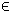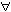## 15GLC Problem

The global mixed-integer nonlinear programming (glc) problem is defined as
 min x
f(x)

s/t
 −∞ < xL ≤ x ≤ xU < ∞ bL ≤ A x ≤ bU cL ≤ c(x) ≤ cU, xjNjI,
(17)
where x, xL, xURn, f(x)R, ARm1 × n, bL,bURm1 and cL,c(x),cURm2. The variables xI, the index subset of 1,...,n, are restricted to be integers.

The following files define a problem in TOMLAB.

File: tomlab/quickguide/glcQG_f.m, glcQG_c.m
```  f:   Function
c:   Constraints
```
The following file illustrates how to solve a constrained global optimization problem in TOMLAB. Also view the m-files specified above for more information.

File: tomlab/quickguide/glcQG.m

Open the file for viewing, and execute glcQG in Matlab.
```% glcQG is a small example problem for defining and solving
% constrained global programming problems using the TOMLAB format.

Name = 'Hock-Schittkowski 59';
u = [75.196    3.8112    0.0020567  1.0345E-5  6.8306    0.030234   1.28134E-3 ...
2.266E-7  0.25645   0.0034604  1.3514E-5  28.106    5.2375E-6  6.3E-8     ...
7E-10     3.405E-4  1.6638E-6  2.8673     3.5256E-5];

x_L = [0 0]';     % Lower bounds for x.
x_U = [75 65]';   % Upper bounds for x.
b_L = []; b_U = []; A = []; % Linear constraints
c_L = [0 0 0];    % Lower bounds for nonlinear constraints.
c_U = [];         % Upper bounds for nonlinear constraints.
x_opt = [13.55010424 51.66018129]; % Optimum vector
f_opt = -7.804226324;              % Optimum
x_min = x_L;      % For plotting
x_max = x_U;      % For plotting
x_0 = [90 10]';   % If running local solver

Prob = glcAssign('glcQG_f', x_L, x_U, Name, A, b_L, b_U, ...
'glcQG_c', c_L, c_U, x_0, ...
[], [], [], [], ...
[], x_min, x_max, f_opt, x_opt);

Prob.user.u = u;
Prob.optParam.MaxFunc = 1500;

Result = tomRun('glcFast', Prob, 1);
%Result = tomRun('glcSolve', Prob, 1);
%Result = tomRun('lgo', Prob, 1);
%Result = tomRun('oqnlp', Prob, 1);```